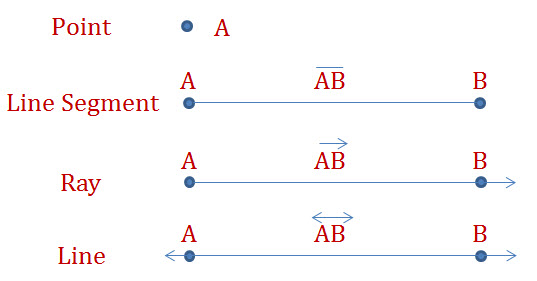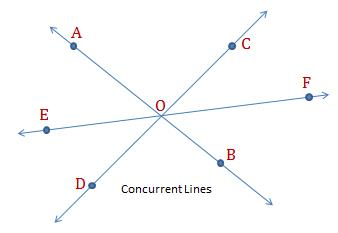Basic Geometrical Concepts

Axioms: The basic facts that are taken for granted, without mathematical proof, are called Axioms. Therefore, the axiom can be used as the premise or starting point for further reasoning or arguments, usually in logic or in mathematics.

Example: A line is composed of infinite points. The halves of equals are equal.

Statement: A statement (or proposition) is a sentence that is either true or false (both not both).

Example: ‘5 is an odd integer’ is a statement. But ‘11 is a cool number’ is not a (mathematical) statement. The sum of the angles of a triangle is 180 degree is a true statement.

Theorem: A statement that requires a proof is called Theorem. A theorem can also be defined as a statement that has been proved on the basis of previously established statements, such as other theorems—and generally accepted statements, such as axioms. A theorem is a logical consequence of the axioms.

Example: The Pythagoras Theorem$a^2+b^2=c^2$

Fundamental Geometrical Terms

Point: An entity that has a location in space or on a plane, but has no extent. In simple term it is like a fine dot denoted by capital letter A, B C etc.Line Segment: Aline segment is a part of a line that is bounded by two distinct end points, and contains every point on the line between its endpoints A and B. The A and B are called its end points. The line segment has a definite length. Line segment is denoted by$\overline{AB}$.

Ray: A line segment AB when extended infinitely in one direction is called a Ray and is denoted by$\overrightarrow{AB}$. A ray only has one end point A. A ray does not have definite length.

Line: A line segment$AB$ when extended infinitely on both sides is called a line and is denoted by$\overleftrightarrow{AB}$. Off course it does not have end points and no definite length. Line can be represented by small letters like$l, m, n$

Incidence Axioms on Lines

1. A line contains infinite number of points
2. Infinite number of lines can be drawn through one point
3. Only one line can be drawn through two given point A and B

Collinear Points: A set of three or more points are called collinear if one line can be drawn through all of them.

Plane: It is a flat surface (two dimensional surface) that extends infinitely in all four directions.

Intersecting lines: Two lines that have a common point are said to be intersecting lines. The common point of the two intersecting lines is call point of intersection.Concurrent Lines: Three of more lines in a plane are said to be concurrent if they all intersect at one pointParallel Lines: Two lines in a plane are said to be parallel if they do not have any point in common. There lines (say l and m) are denoted as$l \parallel m$